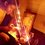# Derivative of peculiar functions

Differentiation is considered an easy topic in calculus. Far more easier than integration, but Some functions are just made to be painful. Today i will be discussing with you guys the derivative of these functions . There are mainly 3 of them $$|x| ,\lfloor x\rfloor$$ and $$\{ x\}$$ . Let's Begin !

$1) \quad |x|$

There are two ways to calculate the derivative of $|x|$ . If you know any other method , please share it in the comments section below.

$\text{Let } v=|x| \implies v^2=x^2 \quad \quad\quad\quad\quad\quad\quad \quad\quad\quad\quad\quad\quad \quad\quad\quad\quad\quad\text{: Alternate :} \\ \text{Take Derivative w.r.t to x on both sides} \quad \quad\quad\quad\quad\quad\quad \quad\quad\quad\quad f(x)=|x|=\sqrt{x^2} \\ 2v\dfrac{dv}{dx}=2x \implies \dfrac{dv}{dx}=\dfrac{x}{v} \quad \quad\quad\quad\quad\quad\quad \quad\quad\quad\quad\quad\quad\quad\quad\quad f^{'}(x)=\dfrac{1}{2\sqrt{x^2}}\cdot2x \\ \boxed{\dfrac{dv}{dx}=\dfrac{x}{|x|}} \quad \quad\quad\quad\quad\quad\quad\quad \quad\quad\quad\quad\quad\quad\quad\quad\quad\quad\quad\quad\quad\quad\quad \boxed{f^{'}(x)=\dfrac{x}{|x|}}$

The Result makes a lot of sense .... if $x>0$, the Derivative is $1$ and if $x<0$, Derivative is $-1$

This is indeed what the graph of $|x|$ tells us!

Now Let's take a general Case of $f(x)=|ax^b+c|^d$, Where $a,b,c,d \in \mathbb R$

$f^{'}(x)=d\cdot|ax^b+c|^{d-1}\cdot\dfrac{d\left(|ax^b+c|\right)}{dx} \\ f^{'}(x)=d\cdot|ax^b+c|^{d-1}\cdot\dfrac{ax^b+c}{|ax^b+c|}\cdot ab\cdot x^{b-1} \\ \boxed{f^{'}(x)=abd\cdot x^{b-1}\cdot|ax^b+c|^{d-2}\cdot{(ax^b+c)}}$

$2) \quad \lfloor x\rfloor$

When floor function or Greatest integer function is applied on a function , the range changes to integral values. We can say that the derivative of the function is $0$ when the function is continuous. The derivative does not exists at integer values of x, because the graph is discontinuous .

For a General $f(x)= \lfloor ax^b+c \rfloor^{d}$, the derivative will always be $\boxed{0}$ .

$3) \quad \{ x \}$

When Fractional part function is applied on a function , the Range changes to $[0,1)$ .

$\{ f(x) \}$ can be written as $f(x)-\lfloor f(x)\rfloor$. Using this property we will find the derivative of $\{ x \}$ . The derivative does not exists at integer values of $x$ , because the graph is discontinuous .

Let $f(x) = \{ x \} = x - \lfloor x \rfloor \\ f^{'}(x)=1 - 0 =1$

For a General $f(x) = \{(ax^b+c)^d\} = (ax^b+c)^d - \lfloor (ax^b+c)^d \rfloor$

$f^{'}(x) = d\cdot(ax^b+c)^{d-1}\cdot abx^{b-1} - 0 \\ \boxed{f^{'}(x) = abd\cdot x^{b-1}(ax^b+c)^{d-1}}$

I Hope you enjoyed this :)Note by Sabhrant Sachan
5 years, 1 month ago

This discussion board is a place to discuss our Daily Challenges and the math and science related to those challenges. Explanations are more than just a solution — they should explain the steps and thinking strategies that you used to obtain the solution. Comments should further the discussion of math and science.

When posting on Brilliant:

• Use the emojis to react to an explanation, whether you're congratulating a job well done , or just really confused .
• Ask specific questions about the challenge or the steps in somebody's explanation. Well-posed questions can add a lot to the discussion, but posting "I don't understand!" doesn't help anyone.
• Try to contribute something new to the discussion, whether it is an extension, generalization or other idea related to the challenge.

MarkdownAppears as
*italics* or _italics_ italics
**bold** or __bold__ bold
- bulleted- list
• bulleted
• list
1. numbered2. list
1. numbered
2. list
Note: you must add a full line of space before and after lists for them to show up correctly
paragraph 1paragraph 2

paragraph 1

paragraph 2

[example link](https://brilliant.org)example link
> This is a quote
This is a quote
    # I indented these lines
# 4 spaces, and now they show
# up as a code block.

print "hello world"
# I indented these lines
# 4 spaces, and now they show
# up as a code block.

print "hello world"
MathAppears as
Remember to wrap math in $$ ... $$ or $ ... $ to ensure proper formatting.
2 \times 3 $2 \times 3$
2^{34} $2^{34}$
a_{i-1} $a_{i-1}$
\frac{2}{3} $\frac{2}{3}$
\sqrt{2} $\sqrt{2}$
\sum_{i=1}^3 $\sum_{i=1}^3$
\sin \theta $\sin \theta$
\boxed{123} $\boxed{123}$

Sort by:

Note: When taking derivatives of continuous and non-differentiable functions, it is important to state the domain of the derivative / the points where the derivative doesn't exist.

This will help prevent mistakes which arise from not realizing that the derivative does not exist, and hence we cannot apply various theorems to it.

E.g. If we want to find the local minimum of $f(x) = |x|$, we cannot differentiate the function and say "no solutions to $f'(x) = 0$ hence no local minimum".

Staff - 5 years, 1 month ago

Really useful, you don't find derivatives of these functions in regular books!

- 5 years, 1 month ago

Yeah, the problem is that in some test questions the domain is not mentioned and I choose "cannot be determined option" but they say that we should stick to the uncommon common sense. -.-

- 5 years, 1 month ago

Really neat work! Loved it. :)

- 4 years, 10 months ago

thank you

- 4 years, 10 months ago

Awesome !

- 5 years, 1 month ago

thanks :)

- 5 years, 1 month ago

Quite useful dude

- 5 years, 1 month ago

That large block of latex full of quads...

- 5 years ago

probably he used an online editor to write the code and pasted it here!

- 4 years, 8 months ago